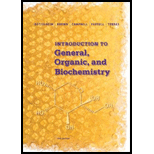# 5-45 An average pair of lungs has a volume of 5.5 L. If the air they contain is 21% oxygen, how many molecules of O 2 do the lungs contain at 1.1 atm and 37°C?### Introduction to General, Organic a...

11th Edition
Frederick A. Bettelheim + 4 others
Publisher: Cengage Learning
ISBN: 9781285869759### Introduction to General, Organic a...

11th Edition
Frederick A. Bettelheim + 4 others
Publisher: Cengage Learning
ISBN: 9781285869759

#### Solutions

Chapter
Section
Chapter 5, Problem 5.45P
Textbook Problem
171 views

## 5-45 An average pair of lungs has a volume of 5.5 L. If the air they contain is 21% oxygen, how many molecules of O2 do the lungs contain at 1.1 atm and 37°C?

Expert Solution
Interpretation Introduction

Interpretation:

Molecules of oxygen in the lungs should be calculated with 1.1 atm and 37 ° C if the air contains 21% oxygen with the average pair of lungs has a volume of 5.5 L.

Concept Introduction:

The ideal gas equation is as follows:

PV=nRT

Here, p is the pressure, v is the volume, n is the number of moles, r is the gas constant and t is the temperature.

Also, number of moles of gas is related to mass and molar mass as follows:

n=mM

Here, m is mass and m is molar mass.

### Explanation of Solution

Given information:

Average pair of lungs has a volume of 5.5 L at 1.1 atm and 37 ° C. The air contains 21% oxygen.

The number of moles of gas can be calculated using the following ideal gas equation:

PV=nRT

Rearranging the equation:

n=PVRT

On putting the values,

n=PVRT

n=(1.1 atm)(5.5 L)(0.082 L atm mol1 K1)(310 K)=0.238 mol

Total mass of gas in the lungs =0

### Want to see the full answer?

Check out a sample textbook solution.See solution

### Want to see this answer and more?

Bartleby provides explanations to thousands of textbook problems written by our experts, many with advanced degrees!

See solution